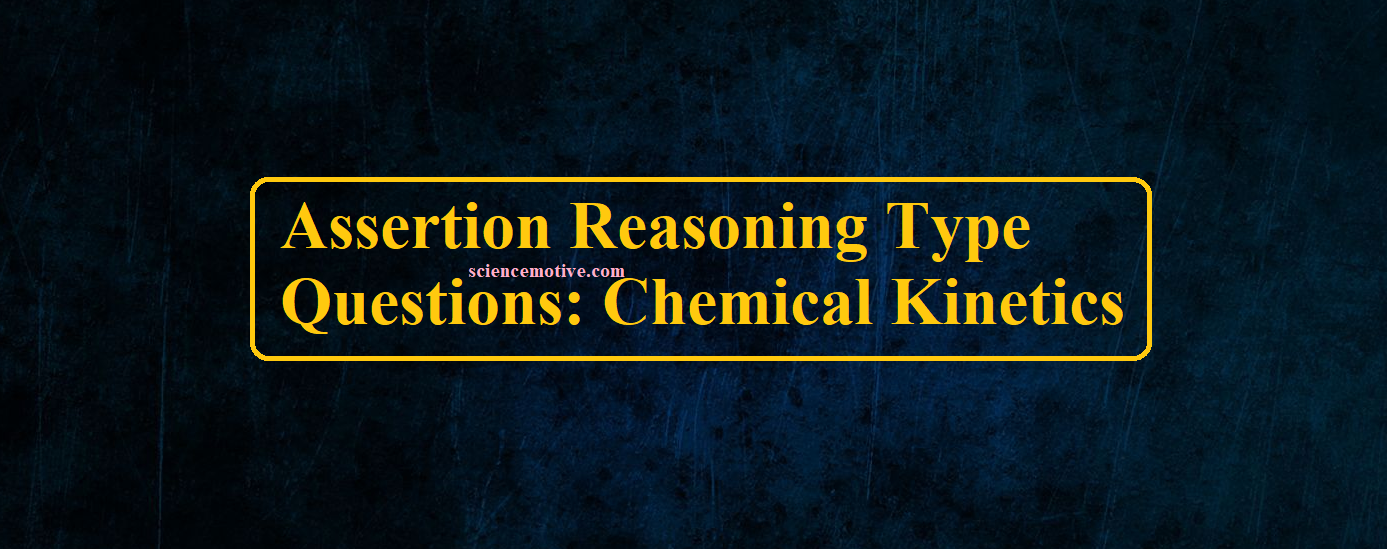# Assertion Reasoning Type Questions: Chemical Kinetics# Assertion Reasoning Type Questions: Chemical Kinetics

Assertion Reasoning Type Questions

Read the statements given as assertion & reason both and choose the correct option as per the following instructions.
(A) if both assertion & reason are correct statements and reason is the correct explanation of assertion.
(B) if both assertion & reason are correct statements and reason is not the correct explanation of assertion.
(C) if the assertion is the correct statement & the reason is an incorrect statement.
(D) if the assertion is incorrect statement and reason is the correct statement.

1. Assertion: The order of reaction can be zero or fractional.
Reason: The order of a reaction cannot be determined from a balanced chemical reaction.
Ans 1. B

2. Assertion: The order and molecularity of a reaction are always the same.
Reason: Order is determined experimentally whereas molecularity by a balanced elementary reaction.
Ans 2. D

3. Assertion: Rate constant of a zero-order reaction has the same unit as the rate of a reaction.
Reason: Rate constant of a zero-order reaction does not depend upon the concentration of the reactant.
Ans 3. A

4. Assertion: In a first-order reaction, the concentration of the reactant is doubled, its half-life is also doubled.
Reason: The half-life of a reaction does not depend upon the initial concentration of the reactant in a first-order reaction.
Ans 4. D

5. Assertion: Average rate and instantaneous rate of a reaction have the same unit.
Reason: Average rate becomes an instantaneous rate when the time interval is too small.
Ans 5. B

6. Assertion: Hydrolysis of methyl ethanoate is a pseudo-first-order reaction.
Reason: Water is present in large excess and therefore its concentration remained constant throughout the reaction.
Ans 6. A

7. Assertion: The order of a reaction can be zero.
Reason: In the case of heterogeneous catalysis, the reaction becomes independent of concentration at a high concentration of the reaction.
Ans 7. A

8. Assertion: The slowest elementary step in a complex reaction decides the rate of the reaction.
Reason: The slowest elementary step always has the smallest molecularity.
Ans 8. C

9. Assertion: A catalyst increases the rate of a reaction.
Reason: The catalyst increases the activation energy which in turn increases the rate of the reaction.
Ans 9. C

10. Assertion: Activation complex for the forward reaction will have lower energy than that for the backward reaction in an exothermic reaction.
Reason: Reactants have greater energy than products for an exothermic reaction.
Ans 10. D

11. Assertion: Increase in temperature increases the rate of reaction.
Reason: More colliding molecules will have energy greater than threshold energy.
Ans 11. A

12. Assertion: The unit of the rate constant is independent of the order of the reaction.
Reason: The power of concentration terms in the rate equation keeps changing with change in order.
Ans 12. D

13. Assertion: Increase in concentration of reactant will not change the rate for a zero-order reaction.
Reason: Rate constant for a zero-order reaction is a constant for a particular initial concentration.
Ans 13. B

14. Assertion: Complex reaction takes place in different steps and the slowest step determines the rate of reaction.
Reason: The order and molecularity of a reaction are always equal.
Ans 14. C

15. Assertion: The rate of reaction increases with an increase in temperature.
Reason: The number of effective collisions increases with an increase in temperature.
Ans 15.  A

16. Assertion: The order of a reaction with respect to any reactant or product can be zero, positive, negative, and fractional.
Reason: The rate of a reaction cannot decrease with an increase in the concentration of a reactant or product.
Ans 16. C

17. Assertion: The rate of a reaction sometimes does not depend on concentration.
Reason: Lower the activation energy faster is the reaction.
Ans 17. B

18. Assertion: For a certain reaction, a large fraction of molecules have energy more than the threshold energy, still the rate of reaction is very slow.
Reason: The colliding molecules must not be properly oriented for effective collisions.
Ans 18. A

Assertion Reasoning Type Questions